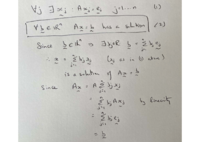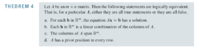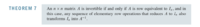# Inverse of a matrix: statement, true or false?

#### HelpNeeder

##### New member
If A is an n x n matrix and Ax = ej is consistent for every j ∊ {1, 2, ..., n}, then A is invertible.

Could someone please help me understand why this statement is true/false?

#### lex

##### Junior Member
It is true. It implies that Ax=b has a solution for all b in $$\displaystyle \mathbb{R^n}$$,
which means A has a pivot in every row, which means A can be transformed into $$\displaystyle \text{I}_n$$ using e.r.o. s (since A is n x n, the pivots must be on the diagonal), therefore A is invertible.

In more detail:Now (2) implies that A has a pivot in every row (by your previous work on Theorem 4):A is n x n, so the pivots are on the diagonals, therefore it can be transformed into $$\displaystyle \text{I}_n$$ by elementary row operations.
Therefore A is invertible.•HelpNeeder